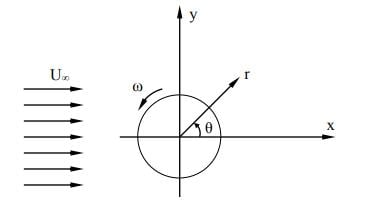## Fluids

•gishnutr
Subscriber

Hi,

I'm working on analyzing fluid flow due to around a rotating cylinder. For the rotating cylinder boundary i ve used Rotational in Moving wall in Momentum tab. I've given speed in rpm as +200 for clockwise rotation of cylinder. also I have entered the x, y coordinates of the center of cylinder in Rotation axis origin tab. Even-though i have got a converged solution my velocity vectors around the rotating cylinder is showing vectors in anti-clockwise direction. when i change angular speed to -ve value the results of velocity vector shows clockwise direction.

•raul.raghav
Subscriber

For 2D Planar simulations, your geometry exists in the XY plane and the Z-axis is oriented out of the computer screen (see image below)If you use the right-hand thumb rule, you'll find that, counter-clockwise (CCW) rotation is +ve and clockwise (CW) rotation is -ve. And if you're monitoring the coefficient of lift (C_l), you'd have a negative C_l value for CCW rotation and a positive C_l value for CW rotation.

So we have,

CCW wall rotation --> (+ve w) --> (-ve C_l)

CW wall rotation --> (-ve w) --> (+ve C_l)

•gishnutr
Subscriber

Hi, Raul

So, i have to use -ve rpm to set the clockwise rotation when axis of rotation is outward to screen.

Could you please tel me how to visually check and verify the rotation direction of cylinder before running the problem.

•raul.raghav
Subscriber

So when you analyze 2D cases in Fluent, the XY plane will lie on the computer screen and the Z axis will be oriented out-of-the-screen towards you. You can place your curled right hand flushed against the computer screen and your thumb will be pointing towards the Z-direction.

So for your question, yes the -ve rpm is set for clockwise rotation. And I'm not sure if there is a way to visually see it before running the problem, but as I suggested earlier you could plot the lift coefficient (C_l) and get a sense of the rotation direction. Refer to the link below to set up the lift coefficient. The tutorial shows the way to set up the drag coefficient, but instead of "Drag" in the list, you should choose "Lift" to get the lift coefficient.

SimCafe: Fluent - Steady Flow Past a Cylinder - Drag Coefficient

Ansys 18.2: Youtube Tutorial Drag Coefficient

•coolboy
Subscriber

Hi, Raul

I have a similar question.

When I set a positive spin rate and monitor the coefficient of lift (C_l),  the lift coefficients are sometimes positive and sometimes negative.

I understand that the direction of lift is (0  1  0), so the lift should always be positive.

Could you please tel me why the lift changes like this.